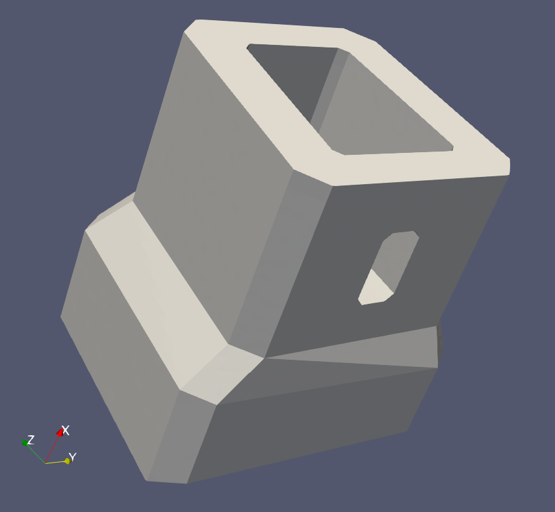top of page
• CHI Consulting Engineers

# Quantifying Rebars in Complex Concrete Structures using Stress Analysis of 3D SolidsNowadays, numerous concrete structures with complex shapes have been built. For these structures, the conventional beam theory usually does not apply since its cornerstone, plane sections remain as planes in deformed shapes, is mostly no longer valid. In the States, the strut-and-tie method (STM) has been used to design complex concrete structures, where the beam theory does not apply. However, the STM was developed for structures with clear load paths. Applying the STM to complex concrete shapes is very challenging, and the results are subjective since they are dependent on engineers’ judgments and experience.

To overcome the above difficulties, the stress-based design of reinforced concrete may be used, and it is often performed in conjunction with linear finite element method (FEM). The linear FEM has been well established and is easy to apply. It does not require subjective engineering judgments and experience, resulting in consistent results. Many load cases can be analyzed efficiently, and their results can be automatically combined or enveloped. Furthermore, the linear FEM reveals locations where rebars are mostly needed, which may not be easily identified by other methods.

Notwithstanding the forgoing, there still existed a big gap between the rebar quantities and 3D stress analysis results. There have been international research and publications on this subject, but there is a lack of commercial software for engineers to use. To bridge this gap, CHI developed an in-house program that can calculate and optimize rebar quantities in three directions using all nine (9) stress components of 3D solid elements. The development of the program went through three phases: Coding; Benchmark study; Application on real projects.

The coding phase involves developing an algorithm for determining the optimal reinforcement quantity using the Python language, as well as a module for converting stress and rebar results into the Paraview software for visualization and further post-processing.

Afterwards, a benchmark study was performed to validate the program. Two cases were conducted: a concrete wall with opening subject to vertical loads; a box section under pure torsion. The first case is a 5-ft thick concrete wall with an opening in its lower potion and vertical loads directly above the opening, as shown in Figure 1. The rebar demands in X direction in Section A are shown in Figure 2, which is based on a 54ksi (0.9*60ksi) rebar strength. The total rebar demand is 3.37in^2 by integration over the entire section, and its centroid of is 12.5 inches from the bottom fiber. To verify this rebar values, a strut-and-tie model (STM) of the same geometry and load was created, as shown in Figure 3. The total rebar demand from the STM is 3.36in^2, a nearly perfect match (3.36/3.37=99.7%) with that from 3D FE analysis.Figure 1. Rebar Demands in X Direction from 3D FE Stress AnalysisFigure 2. Rebar Demands in X Direction in Section AFigure 3. Strut-and-Tie Model for Rebar Demands

A second benchmark study was done using a 5’x5’ concrete box section subject to 1,000 kip-ft torsion, as shown in Figure 4. The contours of calculated longitudinal demands are shown in Figure 5, and the total longitudinal rebar demand in Section A is 9.13in^2. The rebar demand was also calculated in accordance with AASHTO LRFD Bridge Design Specifications, as shown in Figure 6, and it is 9.26in^2, also a nearly perfect match (9.13/9.26=98.6%) with the result from 3D stress analysis.

The results of the two benchmark studies proved the accuracy of CHI’s in-house program of rebar calculation.Figure 4. Concrete Box Section under Pure TorsionFigure 5. Rebar Demands in X Direction of Concrete Box SectionFigure 6. Longitudinal Rebar Demands per AASHTO LRFD

After the calculation program was validated, it has been used on multiple concrete bridge projects, one of which is a signature bridge in US. An example is shown in Figure 7, which consists of a box segment, a solid segment, and an opening. The calculated rebar quantities in both Y and Z directions are shown in Figure 8. The values of CHI’s rebar program are that it not only accurately quantity the rebar demands, but also clearly present the areas/locations of high rebar demands, providing very valuable insights and guidance for rebar detailing.Figure 7. Rebar Demands of a Complex Concrete StructureFigure 8. Rebar Demands in Section A

287 views
bottom of page